New update is available. Click here to update.
Topics

# Normal BST To Balanced BST

Moderate0/80
Average time to solve is 15m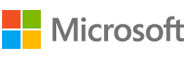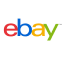## Problem statement

You have been given a binary search tree of integers with ‘N’ nodes. Your task is to convert it into a balanced BST with the minimum height possible.

A binary search tree (BST) is a binary tree data structure that has the following properties.

``````• The left subtree of a node contains only nodes with data less than the node’s data.
• The right subtree of a node contains only nodes with data greater than the node’s data.
• Both the left and right subtrees must also be binary search trees.
``````

A Balanced BST is defined as a BST, in which the height of two subtrees of every node differs no more than 1.

For Example:

``````For the given BST:
``````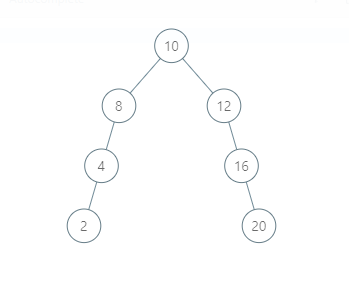``````The modified BST will be:
``````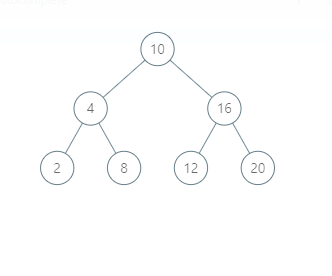Detailed explanation ( Input/output format, Notes, Images )
Sample Input 1:
``````1
10 6 -1 4 -1 -1 -1
``````
Sample output 1:
``````4 6 10
``````
Explanation of Sample output 1:
``````The tree can be represented as follows:
``````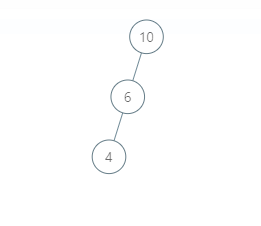``````After converting this tree to balanced BST. It will look like this:
``````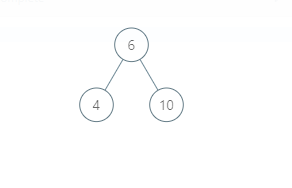``````The inorder traversal of the modified tree will be 4 6 10. Since the inorder is sorted. Hence, it is a valid BST.
``````
Sample Input 2:
``````2
10 5 -1 -1 -1
20 -1 -1
``````
Sample output 2:
``````5 10
20
``````Console